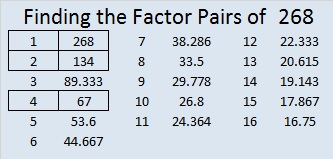# 268 and Level 1

• 268 is a composite number.
• Prime factorization: 268 = 2 x 2 x 67, which can be written (2^2) x 67
• The exponents in the prime factorization are 2 and 1. Adding one to each and multiplying we get (2 + 1)(1 + 1) = 3 x 2  = 6. Therefore 268 has 6 factors.
• Factors of 268: 1, 2, 4, 67, 134, 268
• Factor pairs: 268 = 1 x 268, 2 x 134, or 4 x 67
• Taking the factor pair with the largest square number factor, we get √268 = (√4)(√67) = 2√67 ≈ 16.371.Here is today’s puzzle:Print the puzzles or type the factors on this excel file: 12 Factors 2014-10-20This site uses Akismet to reduce spam. Learn how your comment data is processed.